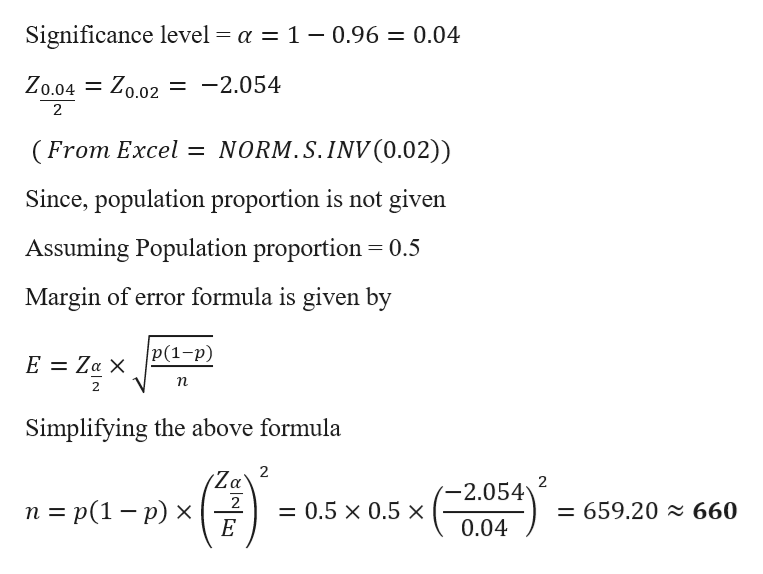# A restaurant would like to estimate the proportion of tips that exceed​ 18% of its dinner bills. Without any knowledge of the population​ proportion, determine the sample size needed to construct a 96​% confidence interval with a margin of error of no more than 4​%to estimate the proportion.The sample size needed is

Question

A restaurant would like to estimate the proportion of tips that exceed​ 18% of its dinner bills. Without any knowledge of the population​ proportion, determine the sample size needed to construct a 96​% confidence interval with a margin of error of no more than 4​%to estimate the proportion.

The sample size needed is
check_circle

Step 1

Given data

Error = 4% = 0.04

Confi...help_outlineImage TranscriptioncloseSignificance level = a = 1 - 0.96 = 0.04 Zo.04 = Zo.02 = -2.054 2 (From Excel = NORM. S.INV (0.02)) Since, population proportion is not given Assuming Population proportion 0.5 Margin of error formula is given by |P(1-р) E Za X п Simplifying the above formula 2 Za 2 -2.054 n %3D p(1 — р) х 2 = 659.20 660 = 0.5 x 0.5 x - Е 0.04 fullscreen

### Want to see the full answer?

See Solution

#### Want to see this answer and more?

Solutions are written by subject experts who are available 24/7. Questions are typically answered within 1 hour.*

See Solution
*Response times may vary by subject and question.
Tagged in

### Other